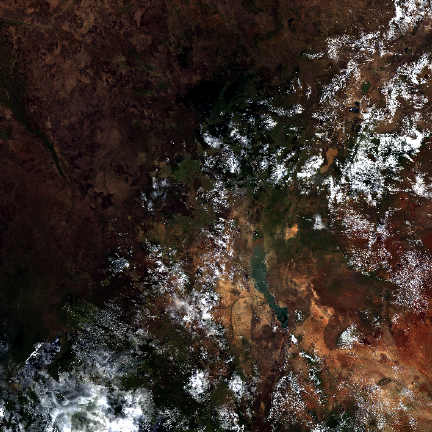# Image exploration¶

## Introduction¶

Now that we have successfully downloaded one MODIS tile, we can use `terra` package to explore and visualize it. Please note that MODIS tiles are distributed in HDF format that may include sub-datasets. The sub-dataset and processing steps might be different for various MODIS collections (e.g. daily scenes, vegetation indices).

Now that we have download some MODIS data, we can explore and visualize it.

First create a SpatRaster object from the file created on the previous page.

```datadir <- file.path(dirname(tempdir()), "_modis")
library(terra)
r <- rast(mf)
r
## class       : SpatRaster
## dimensions  : 2400, 2400, 13  (nrow, ncol, nlyr)
## resolution  : 463.3127, 463.3127  (x, y)
## extent      : 3335852, 4447802, 0, 1111951  (xmin, xmax, ymin, ymax)
## coord. ref. : +proj=sinu +lon_0=0 +x_0=0 +y_0=0 +R=6371007.181 +units=m +no_defs
## sources     : MOD09A1.A2009361.h21v08.061.2021149144347.hdf:MOD_Grid_500m_Surface_Reflectance:sur_refl_b01
##               MOD09A1.A2009361.h21v08.061.2021149144347.hdf:MOD_Grid_500m_Surface_Reflectance:sur_refl_b02
##               MOD09A1.A2009361.h21v08.061.2021149144347.hdf:MOD_Grid_500m_Surface_Reflectance:sur_refl_b03
##               ... and 10 more source(s)
## varnames    : MOD09A1.A2009361.h21v08.061.2021149144347
##               MOD09A1.A2009361.h21v08.061.2021149144347
##               MOD09A1.A2009361.h21v08.061.2021149144347
##               ...
## names       : sur_refl_b01, sur_refl_b02, sur_refl_b03, sur_refl_b04, sur_refl_b05, sur_refl_b06, ...
```

Exercise: Find out at least 5 properties (path, row, date of collection etc) of the MODIS data from the information embedded in the filename.

## Image properties¶

The code below illustrates how you can load HDF files and access image properties of a SpatRaster object.

The coordinate reference system (CRS)

```crs(r)
##  "PROJCRS[\"unnamed\",\n    BASEGEOGCRS[\"Unknown datum based upon the custom spheroid\",\n        DATUM[\"Not specified (based on custom spheroid)\",\n            ELLIPSOID[\"Custom spheroid\",6371007.181,0,\n                LENGTHUNIT[\"metre\",1,\n                    ID[\"EPSG\",9001]]]],\n        PRIMEM[\"Greenwich\",0,\n            ANGLEUNIT[\"degree\",0.0174532925199433,\n                ID[\"EPSG\",9122]]]],\n    CONVERSION[\"Sinusoidal\",\n        METHOD[\"Sinusoidal\"],\n        PARAMETER[\"Longitude of natural origin\",0,\n            ANGLEUNIT[\"degree\",0.0174532925199433],\n            ID[\"EPSG\",8802]],\n        PARAMETER[\"False easting\",0,\n            LENGTHUNIT[\"metre\",1],\n            ID[\"EPSG\",8806]],\n        PARAMETER[\"False northing\",0,\n            LENGTHUNIT[\"metre\",1],\n            ID[\"EPSG\",8807]]],\n    CS[Cartesian,2],\n        AXIS[\"(E)\",east,\n            ORDER,\n            LENGTHUNIT[\"Meter\",1]],\n        AXIS[\"(N)\",north,\n            ORDER,\n            LENGTHUNIT[\"Meter\",1]]]"
```

And the number of cells, rows, columns

```dim(r)
##  2400 2400   13
nrow(r)
##  2400
ncol(r)
##  2400
# Number of layers (bands)
nlyr(r)
##  13
ncell(r)
##  5760000
```

The spatial resolution is about 500 m

```res(r)
##  463.3127 463.3127
```

The layernames tell us what “bands” we have

```names(r)
##   "sur_refl_b01"         "sur_refl_b02"         "sur_refl_b03"
##   "sur_refl_b04"         "sur_refl_b05"         "sur_refl_b06"
##   "sur_refl_b07"         "sur_refl_qc_500m"     "sur_refl_szen"
##  "sur_refl_vzen"        "sur_refl_raz"         "sur_refl_state_500m"
##  "sur_refl_day_of_year"
```

## Plot¶

Now let’s make some simple plots to see if things look reasonable.

```# Create an image RGB composite plot
plotRGB(r, r = 1, g = 4, b = 3)
``````# Disappointing? apply some stretching; see `?plotRGB` for more options
plotRGB(r, r = 1, g = 4, b = 3, stretch="lin")
```Exercise: Create False Color Composite plot using the same data. Hint: try `plotRGB` and specify the bands you need.

Exercise: Save the plots to graphics files. Hint: have a look at `?png`.